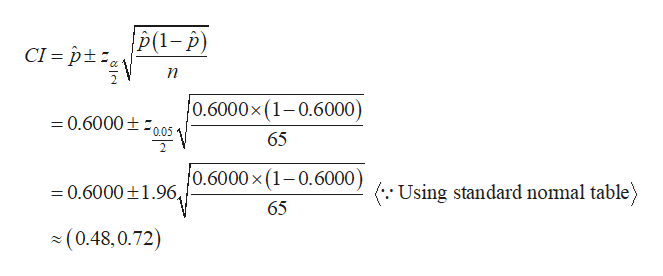# For this problem, carry at least four digits after the decimal in your calculations. Answers may vary slightly due to rounding.In a random sample of 65 professional actors, it was found that 39 were extroverts.(a) Let p represent the proportion of all actors who are extroverts. Find a point estimate for p. (Round your answer to four decimal places.)(b) Find a 95% confidence interval for p. (Round your answers to two decimal places.)lower limit     upper limit

Question
104 views

For this problem, carry at least four digits after the decimal in your calculations. Answers may vary slightly due to rounding.

In a random sample of 65 professional actors, it was found that 39 were extroverts.

(a) Let p represent the proportion of all actors who are extroverts. Find a point estimate for p. (Round your answer to four decimal places.)

(b) Find a 95% confidence interval for p. (Round your answers to two decimal places.)
 lower limit upper limit

check_circle

Step 1

It is given that 39 are extroverts out of 65 professional actors.

Step 2

a)

The point estimate for p is 0.6000 (=39/65).

b)

The 95% confidence ...help_outlineImage TranscriptioncloseCI pt n f0.6000 x (1-0.6000) 0.6000 0.05 65 0.6000 x (1-0.6000) (. Using standard nomal table =0.6000 1.96, 65 (0.48,0.72) fullscreen

### Want to see the full answer?

See Solution

#### Want to see this answer and more?

Solutions are written by subject experts who are available 24/7. Questions are typically answered within 1 hour.*

See Solution
*Response times may vary by subject and question.
Tagged in
MathStatistics